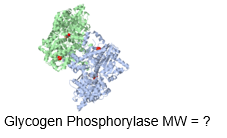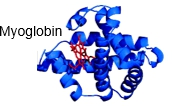## Biochemistry

Learn the toughest concepts covered in Biochemistry with step-by-step video tutorials and practice problems by world-class tutors

4. Protein Structure

# Approximating Protein Mass

1
concept

## Approximating Protein Mass3m
Play a video:
in this video, we're gonna learn how to approximate the mass of a protein. So each amino acid actually has a unique mass, except for just two amino acids. And those two exceptions air losing and is a lucid. And that's because they are ice summers of one another. So they had the same exact chemical formula and the same exact mass. Now, most proteins and nature are quite large, and they range from having several hundreds of amino acids up to several thousands of amino acids. And so calculating the exact mass of a protein with so many amino acids could be a pretty cumbersome, long, drawn out process, especially if we need to take into account the unique masses of the amino acids. And so because it is such a cumbersome process, it's not very likely that your professor wants you to calculate the exact massive a protein. But it is likely that your professor wants you to approximate the massive a protein. But luckily, the massive a protein can be pretty easily approximated with Onley, the total number of amino acid residues. And so when it comes to approximating the massive a protein we need to consider two different factors. The first is the average molecular weight of the 20 free Alfa amino acids, which is 128 g. Promote and molecular weight can be abbreviated with M W or M R for relative molecular weight, which is the same value as the molecular weight, and we're going to treat them as being the same. Now, the key to this first point here is actually the word free. And so recall that free amino acids are separate and independent from one another, and they're not linked via peptide bonds. And so this is the molecular weight, the average molecular weight of the free amino acids. Now, with the second point here, we need to recall that a water molecule and H 20 molecule is lost with the formation of each peptide bond via a dehydration synthesis, reaction toe link and amino acids. And so that means that we need to consider the molecular weight of a water molecule, which is 18 g promote. And so essentially, this makes the average molecular weight of an amino acid residue to be 110 g per mole, and essentially we get this number by taking the difference between the average molecular weight of the free amino acids, minus the molecular weight of a water molecule that's lost with the formation of each peptide bond. So 128 minus 18 gives us 110 and so down below. We have an equation for the approximate massive a protein which is equal to the total number of amino acid residues times the average molecular weight of each amino acid residue, which is 110 and so down below in our example, is asking us to determine the approximate molecular weight of a protein containing amino acids. And so we know that the molecular weight is gonna be equal to the total number of amino acids which is given to us is 200 multiplied by the average molecular weight of ah amino acid residue, which is 110 g per mole. And so if you type this into your calculator 200 times 110 you'll get an answer of 22,000 g per mole, which is of course, equivalent to answer option C here, so we can indicate that see, here is correct. And so this concludes our lesson on approximating the mass of a protein, and we'll be able to get some practice in our next couple of videos, so I'll see you guys there.
2
Problem

What is the approximate M r of the enzyme glycogen phosphorylase, which has 842 amino acid residues?